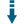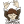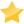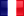# PHP Array Helpers: Perform several array manipulation operationsDownload .zip
 InfoExampleView files (11)Download .zip Reputation Support forum Blog Links
 Last Updated Ratings Unique User Downloads Download Rankings 2021-11-07 (24 days ago)74% Total: 169 All time: 8,732 This week: 241Version License PHP version Categories array-helpers 1.0.3 GNU General Publi... 5.5 Algorithms, PHP 5, Data types
Description Author

This package can perform several array manipulation operations.

It can take a given array and performs several types of unusual array manipulation operations. Currently it can:

- Computing combinations of all the values contained in an array that has nested arrays
- Performing a cross-reference between an array containing keys and an associative array containing associative arrays using the same keys
- Merging two or more arrays together, using their array keys
- Sorting arrays of associative arrays containing the same keys, or arrays of objects having the same properties, providing multiple sort criterion
- Finding items in big arrays using dichotomic search
- Extracting ranges of values from arrays containing integer values
- Converting recursively array items to/from UTF8Performance Level
Name: Classes: Christian Vigh `` 32 packages by Christian VighFrance 55 159 12 in France71 in FranceInnovation awardNominee: 20x

Winner: 3x

Details

# INTRODUCTION

The ArrayHelpers class contains static methods that perform various and completely different operations on arrays. It is to be considered as some kind of swiss-knife and provides the following features :

• Generating combinations of array elements
• Merging array items in a way different from that of the array\_merge() and array\_merge\_recursive() functions
• Performing fast lookups in a sorted array using a dichotomic search
• Recursively encoding/decoding array items to/from UTF8
• And more...

# REFERENCE

## ArrayHelpers::CombinationsOf ( \$array, \$max_results = 10000 ) ;

Takes an array containing values and nested arrays (no more than one level of nesting is authorized) and generates all the possible combinations, each nested array providing alternatives during the generation.

``````
``````

An example will be better than a long description ; suppose that you have the following array :

``````
[ [ 'a', 'b' ], 1, 2, [ 'x', 'y', 'z' ] ]
``````

You want to generate all the possible combinations given the above input, ie, a result such as this one :

``````	'a', 1, 2, 'x'
'a', 1, 2, 'y',
'a', 1, 2, 'z'
'b', 1, 2, 'x',
...
'b', 1, 2, 'z'
``````

The CombinationsOf method will do the work and will return you the following array :

``````
``````

[ [ 'a', 1, 2, 'x' ] [ 'b', 1, 2, 'x' ] [ 'a', 1, 2, 'y' ] [ 'b', 1, 2, 'y' ] [ 'a', 1, 2, 'z' ] [ 'b', 1, 2, 'z' ] ]

``````
``````

For example :

``````	\$result 	=  ArrayHelpers::CombinationsOf ( [ [ 'a', 'b' ], 1, 2, [ 'x', 'y', 'z' ] ] ) ;
print_r ( \$result ) ;

``````

Of course, if you array does not contain nested arrays, the return value will be an array containing only one element, the value you supplied for the \$array parameter. For example :

``````	ArrayHelpers::CombinationsOf ( [ 1, 2, 3, 4 ] )
``````

will return :

``````	[
[ 1, 2, 3, 4 ]
]
``````

The \$max\_results parameter is here to limit the number of items that will be returned by the function, just in case you provided array data which leads to exponential combinations.

Note that :

• Results are computed from left to right
• Currently, only one nesting level can be processed. For example, you cannot supply input values such as :

[ [ 'a', [ 'b', 'c' ] ], 1, 2, [ 'x', 'y', 'z' ] ]

## ArrayHelpers::CrossReference ( \$keys, \$array, &\$missing = [], &\$extra = [], \$case_sensitive = true ) ;

Performs a cross-reference between two arrays. The first array contains key names, and the second one is either an associative array (with key names) or a regular array (with indexes).

The function checks that each element in \$array (identified either by its key or its numeric index) has a correspondance in \$keys.

On output :

• \$missing will contain the elements of \$keys that are not contained in \$array
• \$extra will contain the elements of \$array that are not present in \$keys

The \$case\_sensitive parameter specifies if comparisons on array keys should be case-sensitive or not. This parameter is useless if the \$array parameter is a non-associative array.

The function returns true if the contents of the \$keys array are strictly the same as the array keys of the \$array parameter, or false otherwise.

## InArray ( \$array, \$value, \$case_insensitive = true )

Checks that the specified value is in the specified array. By default, comparisons are not case-sensitive.

This function can be used if you do not have the extension tha contains the iin_array() function (however, I am unable to tell you what is the name of this extension...).

## \$result = ArrayHelpers::MergeAssoc ( \$array [, ...] ) ;

The MergeAssoc()function can be considered as similar to the PHParray\_merge()* function ; however, it operates on array keys rather than on array values.

For example, the following :

``````print_r ( ArrayHelpers::MergeAssoc ( [ 17 = > 1	], [ 17 => 2, 18 => 3 ] ) ) ;
``````

will produce the following output :

``````Array
(
 => 2,
 => 3
)
``````

while the array_merge() function will give :

``````Array
(
 => 1
 => 2
 => 3
)
``````

## \$result = ArrayHelpers::MergeAssocRecursive ( \$array [, ...] ) ;

The MergeAssocRecursive() function is similar to the PHP array\_merge\_recursive() function. However, like the MergeAssoc() function, it operates on array keys rather than on array values.

For example, the following :

``````\$array_1	=  [ 17 => 'value 17 version 1', 18 => [ 'a' => 'value a', 'b' => 'value b version 1' ] ] ;
\$array_2	=  [ 17 => 'value 17 version 2', 18 => [ 'b' => 'value b version 2', 'c' => 'value c' ] ] ;
\$array_3	=  [ 1 => 'value 1', 19 => 'value 19', 20 => 'value 20' ] ;
print_r ( ArrayHelpers::MergeAssocRecursive ( \$array_1, \$array_2, \$array_3 ) ) ;
``````

will give the following result :

``````Array
(
 => value 17 version 2
 => Array
(
[a] => value a
[b] => value b version 2
[c] => value c
)

 => value 1
 => value 19
 => value 20
)
``````

while the array\_merge\_recursive() function will give :

``````Array
(
 => value 17 version 1
 => Array
(
[a] => value a
[b] => value b version 1
)

 => value 17 version 2
 => Array
(
[b] => value b version 2
[c] => value c
)

 => value 1
 => value 19
 => value 20
)
``````

## ArrayHelpers::MultiSort ( &\$array, \$criterias, \$case_sensitive = true ) ;

The MultiSort() function is intended to sort arrays whose values are associative arrays containing the same keys. It allows for specifying multiple sort criterias.

The \$array parameter can either be an array of associative arrays, or an array of objects.

Each element in the \$criteria array specifies a sort criteria, which must reference an array item key in the \$array parameter (if array items are themselves associative arrays) or a property (if array items are objects).

Each value in the \$criterias array must be a boolean value that indicates the sort direction for the associated array key or object property name : true for sorting in ascending order, false for descending order.

On output, the original contents of \$array will be replaced with their sorted contents, while the function will return the number of comparisons performed.

The following examples sorts the \$array parameter on two elements, 'prop1' (in ascending order) and 'prop2' (in descending order) :

``````\$array	=
[
[ 'prop1' => 1 , 'prop2' => 2  , 'prop3' => 10 ],
[ 'prop1' => 17, 'prop2' => 1  , 'prop3' => 11 ],
[ 'prop1' => 1 , 'prop2' => 900, 'prop3' => 12 ],
[ 'prop1' => 17, 'prop2' => 600, 'prop3' => 11 ],
[ 'prop1' => 18, 'prop2' => 1  , 'prop3' => 11 ]
] ;
``````

``````ArrayHelpers::MultiSort ( \$array, [ 'prop1' => true, 'prop2' => false ] ) ;
print_r ( \$array ) ;
``````

It will output :

``````Array
(
 => Array
(
[prop1] => 1
[prop2] => 900
[prop3] => 12
)
 => Array
(
[prop1] => 1
[prop2] => 2
[prop3] => 10
)
 => Array
(
[prop1] => 17
[prop2] => 600
[prop3] => 11
)
 => Array
(
[prop1] => 17
[prop2] => 1
[prop3] => 11
)
 => Array
(
[prop1] => 18
[prop2] => 1
[prop3] => 11
)
)
``````

This also works on arrays of objects, using the same value for the \$criterias array :

``````class TestItem
{
public		\$prop1, \$prop2, \$prop3 ;
``````

``````	public function  __construct ( \$prop1, \$prop2, \$prop3 )
{
\$this -> prop1	=  \$prop1 ;
\$this -> prop2	=  \$prop2 ;
\$this -> prop3	=  \$prop3 ;
}
}
``````

``````\$array	=
[
new TestItem (  1,   2, 10 ),
new TestItem ( 17,   1, 11 ),
new TestItem (  1, 900, 12 ),
new TestItem ( 17, 600, 11 ),
new TestItem ( 18,   1, 11 )
] ;
``````

``````ArrayHelpers::MultiSort ( \$array, [ 'prop1' => true, 'prop2' => false ] ) ;
``````

## \$index = ArrayHelpers::SortedFind ( \$array, \$value ) ;

Returns the index of the element in \$array whose value is equal to the one specified for the \$value parameter.

This function uses a dichotomic search and assumes that the supplied array is already sorted. The complexity of this function is thus O(log2(n)), where n is the number of elements contained in the array (for arrays containing 1 million elements, the number of comparisons will be log2(1000000), so at most 20 comparisons will be required to find the desired element).

It returns boolean false if the specified value was not found, or the index of the specified value in \$array.

The following functions are also available :

• SortedFindLE : Finds the first element in \$array that is less or equal to \$value.
• SortedFindLT : Finds the first element in \$array that is strictly less than \$value.
• SortedFindGE : Finds the first element in \$array that is greater or equal to \$value.
• SortedFindGT : Finds the first element in \$array that is strictly greater than \$value.

## \$result = ToRanges ( \$array ) ;

Extracts ranges of values from an array containing integer elements, and returns an array of 2-elements arrays, which contain in tur the low- and upper-bound of the range.

The following example :

``````\$array	=  [ 1, 2, 3, 4, 5, 10, 11, 12, 20, 21 ] ;
print_r ( ArrayHelpers::ToRanges ( \$array ) ) ;
``````

will display :

``````Array
(
 => Array
(
 => 1
 => 5
)

 => Array
(
 => 10
 => 12
)

 => Array
(
 => 20
 => 21
)

)
``````

Note that the order of elements in \$array has no importance ; you could specify as well :

``````\$array	=  [ 1, 10, 4, 11, 3, 12, 20, 2, 21, 5 ] ;
``````

## \$result = ToRangeString ( \$array, \$range_separator = '-', \$item_separator = ', ' ) ;

Converts an array of integers to a string representing ranges. The following for example :

``````\$a =  [ 1, 3, 4, 2, 6, 22, 21, 20, 12  ] ;
echo ( ArrayHelpers::ToRangeString ( \$a ) ) ;
``````

will display :

``````1-4, 6, 12, 20-22
``````

The parameters are the following :

• \$array (array) : Array to be converted to a range list then to its string representation.
• \$range_separator (array) : tring to be used as a separator between the lower and upper limits of a range. Note that the separator will not be used if the lower and upper limits are equal (only one value will be inserted into the return value).
• item_separator (string) : Separator string to be used between two ranges.

## ArrayHelper::Utf8Encode ( &\$array ) ; ArrayHelper::Utf8Decode ( &\$array ) ;

Recursively encodes/decodes an array. Decoded data is put in the supplied \$array argument.Files
File Role Descriptionexamples (7 files)ArrayHelpers.phpclass Class Class sourceLICENSE Lic. License textNOTICE Data Auxiliary dataREADME.md Doc. DocumentationFiles / examples
File Role DescriptionCombinationsOf.php Example Example scriptCrossReference.php Aux. Auxiliary scriptMergeAssoc.php Example Example scriptMergeAssocRecursive.php Example Example scriptMultiSort.php Example Example scriptSortedFind.php Example Example scriptToRanges.php Example Example script

 All time: 8,732 This week: 241All time Utility: 91%100%83%100%- - 74%177Advertise on this siteSite mapNewsletterStatisticsSite tipsPrivacy policyContact
For more information send a message to `info at phpclasses dot org`.